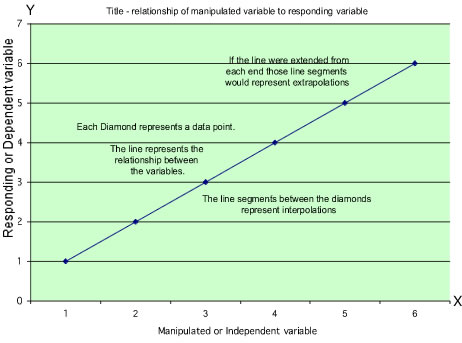# Graphs: Data analysis, variables, relationships, and graphing

A good experiment attempts to control all variables except the variable(s) that are being manipulated to see if a change is observed and a cause and effect relationship can be reasoned.

Variable is a property or condition that can change.
A variable may or may not cause a significant change.

Manipulated variable is the variable the experimenter decides to change to see if there is or is not an effect.

Responding variable is the variable that will change as a result of the change in the manipulated variable. It is observed and measured to determine the quantity or quality of change.

When we plot information on a graph the manipulated variable is always plotted on the X - axis and the responding variable is always plotted on the Y - axis.

Independent variable is another name for manipulated variable. It is independently selected by the experimenter to be manipulated.

Dependent variable is observed by the experimenter and responds to the manipulated, or independent variable, if there is a relationship. Relationships, which can be represented in a chart or graph.

Before deciding to use a chart or graph a person needs to decide if the data is continuous or categorical.

Continuous data is represented on a continuum with infinite possibilities. Temperature for example is represented by whole numbers, decimal numbers, and fractions.

Categorical data is represented by a limited number of examples (discrete, finite, not continuous). While categorical data be continuous or infinite, when it is grouped into categories, it is categorical. Even if there is an other category. Examples: Temperature: hot, medium, or cold. Vehicle color: dark, medium, light. People's favorite foods ... Categories limit possibilities.

Continuous data is best shown on a line graph and categorical in bar, pie, or other kinds of charts.

Extrapolation is a prediction that is made from outside (extra) the data points collected and represented on the graph.

Interpolation is a prediction that is made between the data points (inter, like interstate, between or among states).

Relationship is the way two or more variables, concepts, objects, or people are connected.